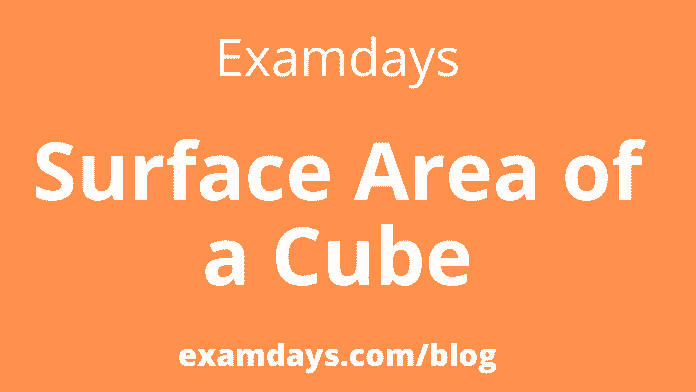# Surface Area of a Cube Formula Definition Solved Examples

0The surface area of ​​a cube is also known as the total area covered by the whole sides of a given cube. Some examples of cubes are Rubik’s cubes, sugar cubes, ice cubes, dice, etc. A cube’s edge describes the length, width, and height connected at the vertices. Six faces of a cube are squares, and the cube’s length, width, and height are equal.

The area of ​the surface is the cube, the sum of the areas of all the faces of the cube that cover it. The formula for surface area is six times the length of the sides of a squared cube. This is denoted by 6a2, where a is the cube’s side length. It is the total surface area. Also, learn about the volume of a cube. When a cube is placed in a three-dimensional space, the area occupied by the sides of the cube in that space is called the surface area of ​​the cube.

## Surface Area of Cube

By definition of the surface area of ​​a given cube, if the total surface area is equal to the sum of the areas for all the faces of the cube. Since a cube has six faces, the entire surface area of ​​the cube is equal to the sum of all six faces of a cube.

So, the surface of the cube is square. Therefore, the area of ​​each face of a cube is equal to the square of the edge.

• Thus, the area of one face = a2
•  total six faces of the cube.
• Therefore TSA of cube is = a2 + a2 + a2 + a2 + a2 +a2
• TSA of a cube is = 6a2

A cube has an ‘n’ number of square units. Hence the area covered by these square units on the cube’s surface is the surface area. Cube has six faces.

### Surface Area of a Cube Formula:

From the definition of the cube we discussed above, we know that a cube has 6 square faces. Consider a cube whose edge length is denoted by the letter ‘a’.

• From the formula area of ​​square = side2 = a2, we know.
• Total surface area cube formula = six × (area of ​​each side)
• = 6 × a2 = 6a2 square unit.

## What is the Surface Area of Cube?

Surface area is a sum of the area of ​​the bases and the area of ​​the lateral surfaces of the cube. All six faces of a cube are made up of squares of the exact dimensions; a cube’s total surface area is six times the surface area of ​​one face. It is measured as a “number of square units”. There are two types of the surface area of ​​a cube. The units of the surface area of ​​a cube and the total surface area of ​​a cube are measured in square units, i.e., m2, cm2, etc. A cube has two types of surface area.

• Total Surface Area of Cube
• Lateral Surface Area of Cube

### Total Surface Area of Cube Formula

The surface area of ​​the cube refers to the area of ​​all sides of the cube. To find the cube’s total surface area, the sum of the areas of all sides is required. The lateral area is the area of ​​a square. The total surface area of ​​the cube is 6 square meters giving the total surface area of ​​the cube.

Lateral Surface Area of Cube

The lateral surface of a cube represents the area of ​​its lateral sides; The base and top of the cube are not included when solving for the lateral surface area of ​​the cube. A cube has four lateral sides of a side square. So, the area of ​​the square is four times the lateral surface area of ​​the cube.

What is the formula for the surface area of a cuboid?

If l is the length, b is the breadth, and h is the height of the cuboid;

Lateral surface area of the cuboid formula = 2h(l+b)

The total surface area of the cuboid formula = 2(lb+bh+lh)

Conclusion

Learn how the formula is derived from the properties of a cube and used to calculate its surface area. Focus on how this principle is used in the solved examples to understand the concept better.

Join Examdays Telegram

For more details about the Telegram Group, Click the Join Telegram below button.Join Membership

In case of any doubt regarding Telegram, you can mail us at [email protected].

This site uses Akismet to reduce spam. Learn how your comment data is processed.